# Optimisation playground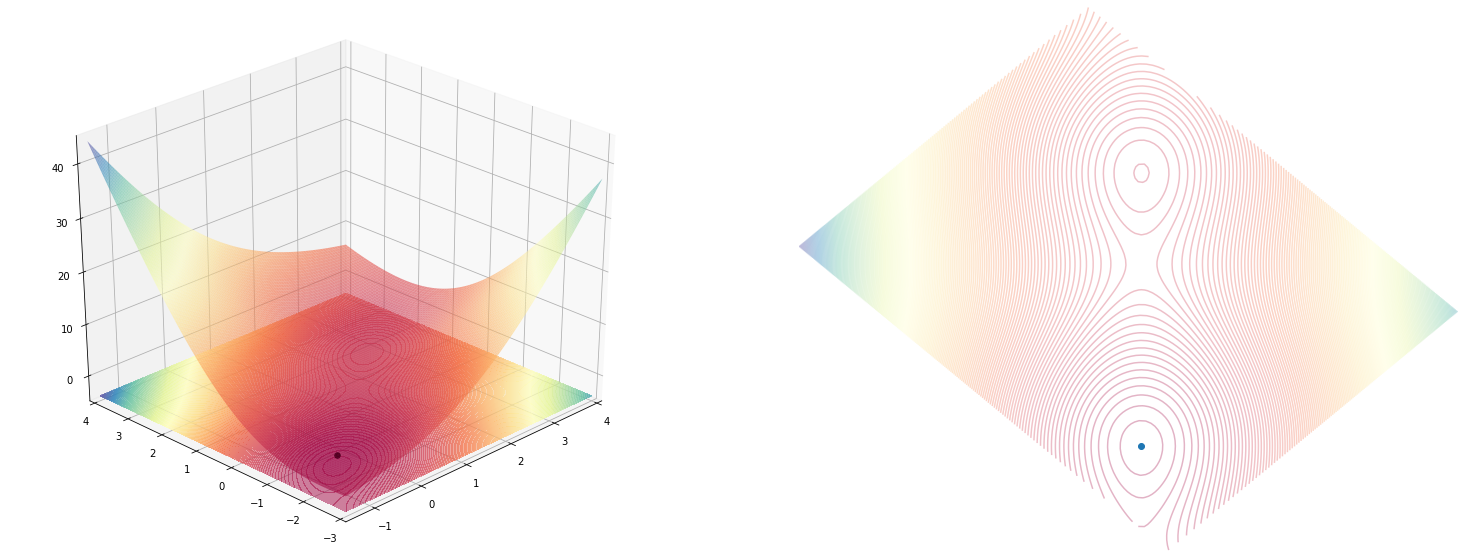# Goal

I’ve just stumbled upon this wiki page which describes optimization methods that can be used for optimizing functions or (or programs) where you don’t know or is hard to compute a derivative for it.

I plan to implement some optimizers from that page and see how they work, but before doing that I realized that I also need a simulation environment where I can see how the optimization is progressing.

In the end, the simulation looks something like this.

Code
import matplotlib.animation as animation
from IPython.display import HTML, display

x, y = [0.], [0.]

def sgd_update(x, y, learning_rate=0.01):
x = x - learning_rate * d_x
y = y - learning_rate * d_y
return x, y

def single_frame(i, _ax_3d, _ax_2d):
_ax_3d.clear()
_ax_2d.clear()
angle = 225
_x, _y = sgd_update(x[-1], y[-1], learning_rate=0.01)
x.append(float(_x)), y.append(float(_y))

plot_function(function, fig=fig, ax_3d=_ax_3d, ax_2d=_ax_2d, angle=angle)
_ax_2d.scatter(*rotate(np.array(x), np.array(y), angle=angle), c=np.arange(len(x)), cmap='binary')

_ax_2d.plot()

function = himmelblau()

fig = plt.figure(figsize=(13,5))
fig, ax_3d, ax_2d = plot_function(function, fig=fig)
fig.tight_layout()

frames = 20
animator = animation.FuncAnimation(fig, single_frame, fargs=(ax_3d, ax_2d), frames=frames, interval=250, blit=False)
display(HTML(animator.to_html5_video()))
plt.close();


And starting from a different point..

Code
import matplotlib.animation as animation
from IPython.display import HTML, display

def single_frame(i, optimisation, _ax_3d, _ax_2d, angle):
_ax_3d.clear()
_ax_2d.clear()

x, y = optimisation.update()
x, y = np.array(x), np.array(y)

plot_function(optimisation.function, angle=angle, fig=fig, ax_3d=_ax_3d, ax_2d=_ax_2d)
_ax_2d.scatter(*rotate(x, y, angle=angle), c=np.arange(len(x)), cmap='cool')
_ax_3d.scatter3D(x, y, optimisation.function(x, y), c=np.arange(len(x)), cmap='cool')

_ax_2d.plot()

angle=225
optimisation = optimize(himmelblau())\
.using(sgd(step_size=0.01))\
.start_from([-0.5, -0.5])\

fig = plt.figure(figsize=(13,5))
fig, ax_3d, ax_2d = plot_function(optimisation.function, fig=fig, angle=angle)
fig.tight_layout()

frames = 20
animator = animation.FuncAnimation(fig, single_frame, fargs=(optimisation, ax_3d, ax_2d, angle), frames=frames, interval=250, blit=False)
display(HTML(animator.to_html5_video()))
plt.close()


This blog post mainly documents the exploration I did and experiments I made that lead to the final form of the simulation environment.

# Test-ground

Optimizations algorithms (and their implementation’s) performance are usually showcased on some known mathematical functions with interesting (and hard) shapes. Some examples:

• Rosenbrock banana function $f(x_1, x_2, x_3, .., x_n) = \sum_{i=1}^{n-1}(100(x_{i+1}-x_i^2)^2+(1-x_i)^2$• Himmelblau’s function $f(x, y) = (x^2+y-11)^2 + (x+y^2-7)^2$At the same time, an optimization algorithm, starts from some where (some from multiple places all at once), and iteratively progress to the final result. So there is a trace element to this environment to see how things are moving along.

# Starting off with Himmelblau’s function

I’ll start with just replicating one random function from that list (Himmelblau) and implement if in pure numpy.

This function will be used to experiment different visualization aspects of it, but in the end we will want to substitute it with any other function we wish to use.

## Numpy implementation

Following the given definition above: $$f(x, y) = (x^2+y-11)^2 + (x+y^2-7)^2$$ the numpy code should be quite simple to write.

import numpy as np
def himmelblau(x: np.ndarray, y: np.ndarray) -> np.ndarray:
return (x**2+y-11)**2 + (x+y**2-7)**2

himmelblau(0, 1)

136


## Drawing the shape (contour) of the function

Now that we have an example function implemented, let’s write the plotting code that shows the 2D contour of it.

This is a 2D function (and we will only deal with 2D functions in our simulation) and since for every $(x,y)$ point we also have the evaluation of the function $f(x,y)$ what we actually need to represent is a $(x, y, f(x,y))$ triple.

In plain 2D you can’t normally plot this kind of information (since you only have 2 dimensions) but you can artificially add a new (3rd) dimension by using colors.

The idea is that if you have some colormap like these,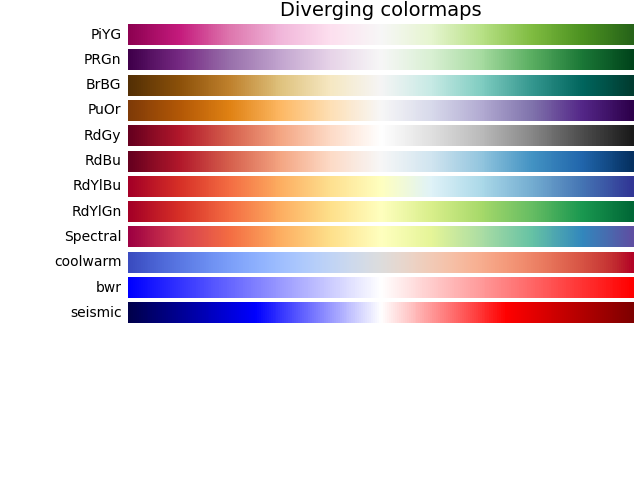you can replace the $f(x, y)$ value by the color you’ve got in that colormap. This will result in a contour plot. You can find a similar idea in other kind of plots like heat-maps or choropleth.

I’m no visualization expert, quite the opposite, so some things I say may be inaccurate, debatable or plain false. In such cases please leave a comment and I’ll try my best to correct this post.

### 2D Contour Plot

We can visualize the contour of any function, by using the plt.contourf function from matplolitb.

This function requires from us a triple of points in (x, y, z) form, which it will use to interpolate the shape of the function. This means that we need lots of probing points in the $(x,y)$ domain for which to evaluate $f(x, y)$.

What you usualy do in this instance is to create a mesh of points, a 2d grid of points, like the intersections of lines on a chess board.

Numpy provides a way of creating these mesh values by using the np.meshgrid function in conjunction with np.linspace.

xx, yy = np.meshgrid(
np.linspace(-5, 5),
np.linspace(-5, 5)
)

xx.shape, yy.shape

((50, 50), (50, 50))


The result of the above function is list of (50, 50) points for the x coordinate and (50, 50) points for the y coordinate. If you’d plot them on a scatter-plot, this is how they would look like.

plt.scatter(xx, yy)
plt.xlim(-1, 1)
plt.ylim(-1, 1)

(-1.0, 1.0)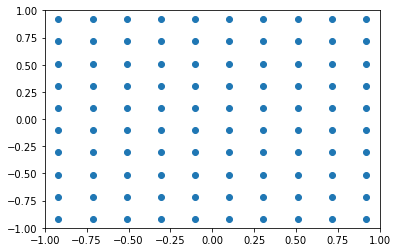The first few values of the yy variable show how it is composed. It’s repeated values on the first axis (i.e. rows are equal among them), and linearly spaced values (between the maximum and minimum) on the second axis (i.e. columns).

yy[:3, :3]

array([[-5.        , -5.        , -5.        ],
[-4.79591837, -4.79591837, -4.79591837],
[-4.59183673, -4.59183673, -4.59183673]])


The xx variable is the opposite of yy. You have equal columns (second axis) and linearly spaced values on the rows (first axis).

xx[:3, :3]

array([[-5.        , -4.79591837, -4.59183673],
[-5.        , -4.79591837, -4.59183673],
[-5.        , -4.79591837, -4.59183673]])


Matplotlib has a function called contourf that will print our contours. There are some details in the code bellow, regarding the color normalization, the color map (cmap) and the number of levels we want contours for which I won’t explain but are easy to understand.

Code
import numpy as np
from mpl_toolkits import mplot3d
from matplotlib import pyplot as plt
import matplotlib.colors as colors

xx, yy = np.meshgrid(
np.linspace(-5, 5, num=100),
np.linspace(-5, 5, num=100)
)
zz = himmelblau(xx, yy)

plt.contourf(xx, yy, zz, levels=1000, cmap='Spectral', norm=colors.Normalize(vmin=zz.min(), vmax=zz.max()))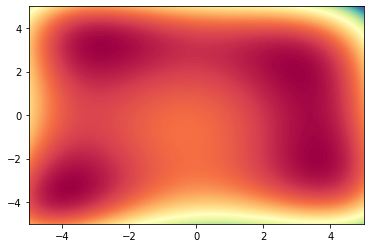### 3D shape

Since this is a 2D function (x, y) and the evaluation of it is a 3D dimension, we should really plot it in 3D.

Since matplotlib wasn’t specifically designed initially for 3D plots, the 3D add-on (included in the default package) is somewhat of a patch over the 2D machinery.

In any case, it was simple enough to print the 3D shape of out plot, as you can see bellow.

Code
import matplotlib.colors as colors

plt.figure(figsize=(13, 10))
ax = plt.axes(projection='3d')
ax.plot_surface(xx, yy, zz, cmap='Spectral', rcount=100, ccount=100, norm=colors.Normalize(vmin=zz.min(), vmax=zz.max()))
ax.contourf(xx, yy, zz, levels=100, zdir='z', offset=np.min(zz)-100, cmap='Spectral', norm=colors.Normalize(vmin=zz.min(), vmax=zz.max()))

# ax.view_init(50, 95)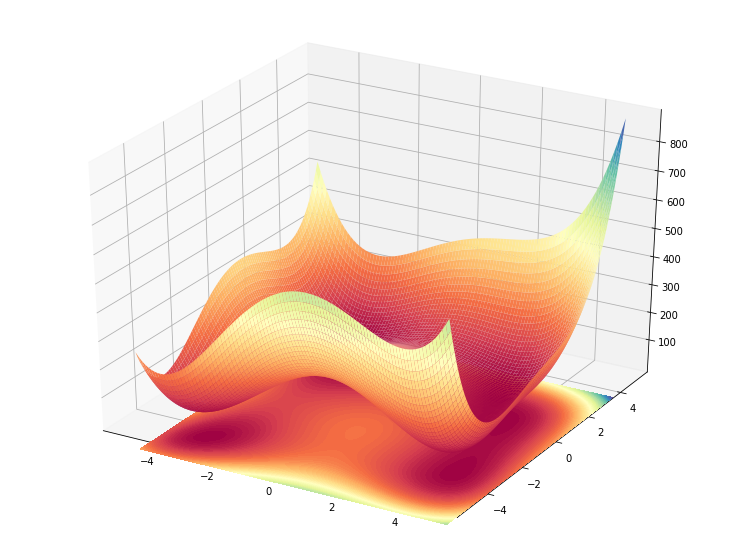On the other hand, some other (maybe reasonable) stuff I tried to accomplish in 3D weren’t so easy, as you can see further down bellow..

## Making an animation of the function

Visualizing a 3D function by a static image is not that great. What would be great instead is if we could somehow see it in motion, rotating the graph in a 360 degree animation. In this way we could better understand the shape we are dealing with.

The two code snippets bellow quickly sketch how to use the matplotlib.animation package on a 3D plot.

Code
def single_frame(i, ax):
ax.clear()
ax.view_init(45, (i % 36) * 10 )
ax.plot_surface(xx, yy, zz, cmap='Spectral', rcount=100, ccount=100, norm=colors.Normalize(vmin=zz.min(), vmax=zz.max()))
ax.contourf(xx, yy, zz, levels=300, offset=np.min(zz)-100, cmap='Spectral', norm=colors.Normalize(vmin=zz.min(), vmax=zz.max()), alpha=0.5)
ax.scatter3D(min_coordinates[:, 0], min_coordinates[:, 1], himmelblau(min_coordinates[:, 0], min_coordinates[:, 1]), marker='.', color='black', s=60, alpha=1)
ax.plot()

plt.figure(figsize=(8, 5))
ax = plt.axes(projection='3d')
single_frame(10, ax)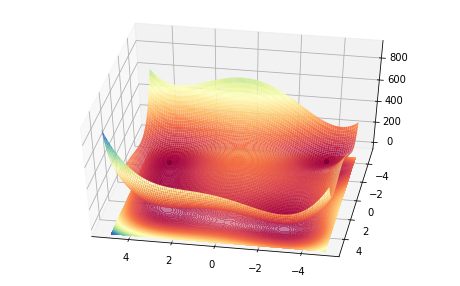import matplotlib.animation as animation
from IPython.display import HTML, display

fig = plt.figure(figsize=(13, 10))
ax = plt.axes(projection='3d')

single_frame(0, ax)
fig.tight_layout()

frames = 36
animator = animation.FuncAnimation(fig, single_frame, fargs=(ax,), frames=frames, interval=100, blit=False)
display(HTML(animator.to_html5_video()))
plt.close()